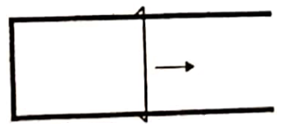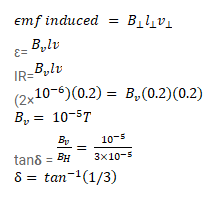# Figure shows a metallic wire of resistance

Question:

Figure shows a metallic wire of resistance $0.20 \Omega$ sliding on a horizontal, U-shaped metallic rail. The separation between the parallel arms is $20 \mathrm{~cm}$. An electric current of $2.0 \mu \mathrm{A}$ passes through the wire when it is slid at the rate of $20 \mathrm{~cm} / \mathrm{s}$. If the horizontal component of the earth's magnetic field is $3.0 \times 10^{-5} \mathrm{~T}$, calculate the dip at the place.Solution: